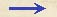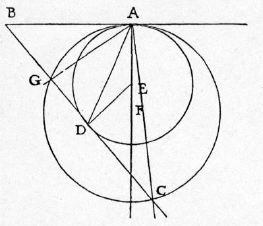Discorsi Propositions 2/32-th-21 Discorsi Proposition2/32-th-21THEOREMA XXI, PROPOSITIO XXXII. THEOREM XXI, PROPOSITION XXXIISi in horizonte sumantur duo puncta, et ab altero ipsorum quaelibet linea versus alterum inclinetur, ex quo ad inclinatam recta linea ducatur, ex ea partem abscindens aequalem ei quae inter puncta horizontis intercipitur, casus per hanc ductam citius absolvetur quam per quascunque alias rectas ex eodem puncto ad eandem inclinatam protractas. If in a horizontal line any two points are chosen and if through one of these points a line be drawn inclined towards the other, and if from this other point a straight line is drawn to the inclined line in such a direction that it cuts off from the inclined line a portion equal to the distance between the two chosen points on the horizontal line, then the time of descent along the line so drawn is less than along any other straight line drawn from the same point to the same inclined line. In aliis autem, quae per angulos aequales hinc inde ab hac distiterint, casus fiunt {20} temporibus inter se aequalibus. Sint in horizonte duo puncta A, B, et ex B inclinetur recta BC, in qua ex termino B sumatur BD, ipsi BA aequalis, et iungatur AD: dico, casum per AD velocius fieri quam per quamlibet ex A ad inclinatam BC productam. Ex punctis enim A, D {254} ad ipsas BA, BD perpendiculares ducantur AE, DE, se se in E secantes: et quia in triangulo aequicruri ABD anguli BAD, BDA sunt aequales, erunt reliqui ad rectos DAE, EDA aequales; ergo, centro E, intervallo EA, descriptus circulus per D quoque transibit, et lineas BA, BD tanget in punctis A, D. Et cum A sit terminus perpendiculi AE, casus per AD citius absolvetur quam per quamcunque aliam ex eodem termino A usque ad lineam BC ultra circumferentiam circuli extensam: quod erat primo ostendendum. Quod si, extenso perpendiculo AE, in eo sumatur quodvis centrum F, et secundum intervallum FA circulus AGC describatur, tangentem lineam in punctis G, C secans, iunctae AG, AC per angulos aequales a media AD,{10} ex ante demonstratis, dirimentur; et per ipsas, lationes temporibus aequalibus absolventur, cum ex puncto sublimi A ad circumferentiam circuli AGC terminentur. Along other lines which make equal angles on opposite sides of this line, the times of descent are the same. Let A and B be any two points on a horizontal line: through B draw an inclined straight line BC, and from B lay off a distance BD equal to BA; join the points A and D. Then, I say, the time of descent along AD is less than along any other line drawn from A to the inclined line BC.From the point A draw AE perpendicular to BA; and from the point D draw DE perpendicular to BD, intersecting AE at E. Since in the isosceles triangle ABD, we have the angles BAD and BDA equal, {254} their complements DAE and EDA are equal. Hence if, with E as center and EA as radius, we describe a circle it will pass through D and will touch the lines BA and BD at the points A and D. Now since A is the end of the vertical line AE, (Condition 2/06-th-06) (Condition 2/02-th-02-cor2) the descent along AD will occupy less time than along any other line drawn from the extremity A to the line BC and extending beyond the circumference of the circle; which concludes the first part of the proposition. If however, we prolong the perpendicular line AE, and choose any point F upon it, about which as center, we describe a circle of radius FA, this circle, AGC, will cut the tangent line in the points G and C.Draw the lines AG and AC which will according to the preceding lemma, deviate by equal angles from the median line AD.The time of descent along either of these lines is the same, since they start from the highest point A, and terminate on the circumference of the circle AGC.Discorsi Propositions 2/32-th-21 Discorsi Proposition2/32-th-21